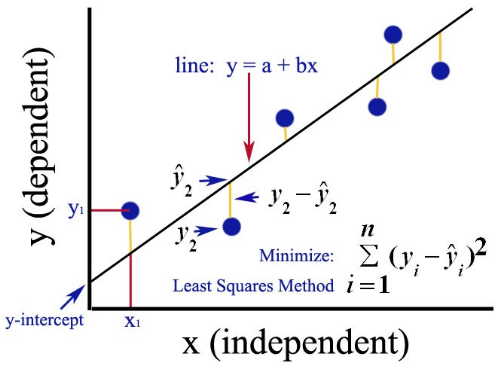# Ordinary Least Square (OLS) Method for Linear RegressionThis post is about the ordinary least square method (OLS) for simple linear regression. If you are new to linear regression, read this article for getting a clear idea about the implementation of simple linear regression. This post will help you to understand how simple linear regression works step-by-step. The simple linear regression is a model with a single regressor (independent variable) x that has a relationship with a response (dependent or target) y that is

This post is about the ordinary least square method (OLS) for simple linear regression. If you are new to linear regression, read this article for getting a clear idea about the implementation of simple linear regression. This post will help you to understand how simple linear regression works step-by-step.

The simple linear regression is a model with a single regressor (independent variable) x that has a relationship with a response (dependent or target) y that is a

y = β0 + β1 x + ε — — — — — — — — — — (1)

Where β0: intercept

β1: slope (unknown constant)

ε: random error component

This is a line where y is the dependent variable we want to predict, x is the independent variable, and β0 and β1 are the coefficients that we need to estimate.

Estimation of β0 and β1 :

The OLS method is used to estimateβ0 and β1. The OLS method seeks to minimize the sum of the squared residuals. This means from the given data we calculate the distance from each data point to the regression line, square it, and the sum of all of the squared errors together.## Linear Regression For Data Science

Linear regression is commonly used to quantify the relationship between two or more variables. It is also used to adjust for confounding.

## Baby Steps Towards Data Science: Multiple Linear Regression in Python

How to implement multiple linear regression and interpret the results. Source code and interesting basketball player dataset has been provided.

## Exploratory Data Analysis is a significant part of Data Science

Data science is omnipresent to advanced statistical and machine learning methods. For whatever length of time that there is data to analyse, the need to investigate is obvious.